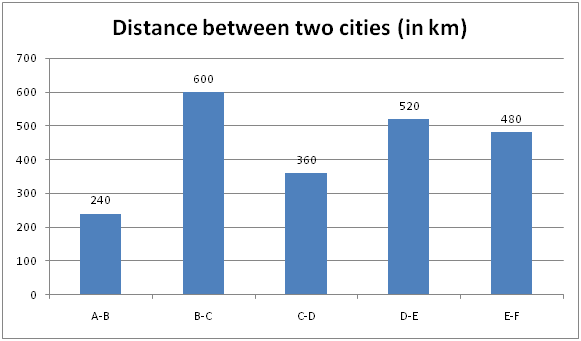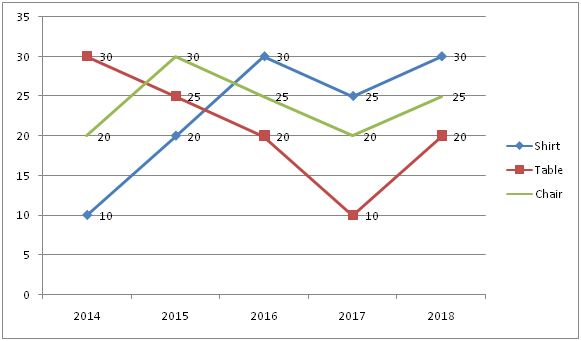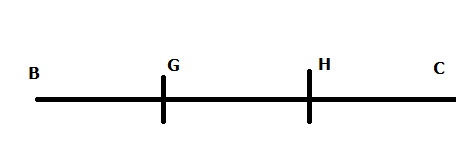# SBI PO Quantitative Aptitude Questions 2019 (Day-15) High Level New Pattern

SBI PO 2019 Notification is about to come and it is the most awaited exam among the aspirants. We all know that new pattern questions are introducing every year in the SBI PO exam. Further, the questions are getting tougher and beyond the level of the candidate’s expectations.

Our IBPS Guide is providing High-Level New Pattern Quantitative Aptitude Questions for SBI PO 2019 so the aspirants can practice it on a daily basis. These questions are framed by our skilled experts after understanding your needs thoroughly. Aspirants can practice these high-level questions daily to familiarize with the exact exam pattern. We wish that your rigorous preparation leads you to a successful target of becoming SBI PO.

[WpProQuiz 5052]

### Click Here for SBI PO Pre 2019 High-Quality Mocks Exactly on SBI Standard

Direction (1 – 5): Study the following information carefully and answer the given questions

The given bar graph shows the distance between two different cities.1) A car travelled from A to B. After the travelling the part of the distance the car developed the engine problem and proceeds at 75% of the former speed and arrived at B in 25 minutes late and had problem developed 20km later the car could have arrived 5 minutes sooner. Find the distance the car travelled by without any problem?

a) 140km

b) 220km

c) 180km

d) 200km

e) None of these

2) The Car start from D to C with the speed of 30 kmph and Bus start from C to D with speed of 10kmph. Car started the journey two hours earlier than Bus to meet each other. If they meet each other at a place S somewhere between C and D, then find the distance between S and D?

a) 265 km

b) 275 km

c) 285 km

d) 295 km

e) None of these

3) Train S starts from station D at 7.45 am and goes towards E and train T starts from E 30 min after train S towards D and they meet at 1.15 pm. If Train T speed is 150% of the speed of train S, then find the speed of train T?

a) 80 kmph

b) 60 kmph

c) 40 kmph

d) 20 kmph

e) None of these

4) Three person-S, T and U travel from B to C. They have a cycle which can carry only two persons. Cycle can be driven only by S and others walk at 30kmph. All of them start their journey from B simultaneously and are required to reach C at the same time. If the speed of cycle is 120kmph, then what is the shortest possible time in which all three can reach C at the same time?

a) 9(2/7) hours

b) 7(2/7) hours

c) 11(2/7) hours

d) 5(2/7) hours

e) None of these

5) A person travel bike and car from E to F. The ratio of the speed of bike to car is 4:5 and the total time taken by bike and car is 12 hours, then what is the speed of car?

a) 90 kmph

b) 100 kmph

c) 80 kmph

d) 60 kmph

e) None of these

Direction (6 – 10): Study the following information carefully and answer the given questions:

The given line graph shows the percentage of discount of three different product in 5 different years.6) If the ratio of the marked price of shirt, table and chair in all the years is 2:3:1. The sum of the selling price of shirt in 2014 and 2015 together is approximately what percent of the sum of the selling price of Table in 2014 and chair in 2016?

a) 115%

b) 119%

c) 123%

d) 142%

e) 169%

7) In 2017, the sum of the selling price of table and chair is Rs.1000. If the sum of the marked price of table and chair in 2017 is Rs.1200, then the marked price of the table is what percent of the marked price of chair in 2017?

a) 50%

b) 40%

c) 60%

d) 30%

e) None of these

8) The selling price of Shirt, Table, Chair and Laptop in 2018 is Rs.700, Rs.2000, Rs.900 and Rs.9900 respectively. If overall discount of 2018 by selling all the product is 10%, then find the discount amount of laptop?

a) Rs.400

b) Rs.500

c) Rs.600

d) Rs.800

e) None of these

9) If the ratio of the cost price of Table and Chair in 2016 is 3:4 and the marked price is 20% and 25% above the cost price of Table and chair in 2016 respectively. If the sum of the loss earned selling them is Rs.185, then what is the difference between the marked price of Table and chair?

a) Rs.600

b) Rs.800

c) Rs.500

d) Rs.700

e) None of these

10) If the marked price of shirt in 2015 is Rs.800 and also the shopkeeper earned 25% profit. If no discount is given, then what is the profit percentage?

a) 25%

b) 75%

c) 48%

d) 42%

e) None of these

Direction (1-5) :

Speed of the car=S kmph

20/(75/100*S)-20/S=5/60

80/3S-20/S=5/60

(80-60)/3S=5/60

20*60=15S

S=80kmph

75% of the speed=80*75/100=60kmph

Total time=240/80=3 hours

x/80+(240-x)/60=3 hours 25 minutes

x/80+(240-x)/60=3+(5/12)

3x+240*4-4x=41/12*240

-x=820-960

X=140km

Car travelled by two hours=2*30=60 km

Remaining distance=360-60=300 km

Meeting time=300/(30+10)=7.5 hours

Total time= (2+7.5)=9.5 hours

Distance covered by Car=30*9.5=285 km

Distance between S and D=285 km

Train S speed=x

Train T speed=150/100*x=3x/2

Train S started 30 minutes before=30/60*x=x/2km

Remaining distance=520-x/2

Total time=(1.15-8.15)=5 hours

(520-x/2)/(x+3x/2)=5

(1040-x)/5x=5

1040-x=25x

1040 = 26x

X=40

Train T speed=3*40/2=60 kmphS starts from B.

S has to take off T on his cycle, take him up to the certain distance drop him (H) and return for U but already U starts walking and reached the point G. S pick up U at the point G and travel towards C.

S travelled=BH+GH

U=BG (distance)

S=120kmph

U=30kmph

S=4*U

BH+HG=4*BG

(BG+GH)+HG=4*BG

BG+2GH=4*BG

3BG=2GH

GH=3/2(BG)

Same time T reaches C from H walking at 30kmph and S and U reach C using cycle.

Now S=HG+GC

T=HC

Speed of T=30kmph

Speed of S=120kmph

S = 4 * T

HG+GC=4*HC

HG+GH+HC=4*HC

2GH=3HC

2 *(3/2*BG) = 3HC

3BG=3HC

BG=HC

BG+GH+HC=600km

BG+3/2*BG+BG=600

2BG+3BG+2BG=600*2

7BG=1200

BG=1200/7

GH=3/2*1200/7=1800/7

HC=1200/7

Required time=(BH+HG+GC)/120

=[(BG+GH)+HG+GH+HC]/120

=BG+3HG+HC/120

T=((1200/7)+(3*1800/7)+1200/7)/120

T=7800/7*120=65/7=9(2/7) hours

480/4x+480/5x=12

120+96=12x

X=18 kmph

Speed of car=5*18=90 kmph

Direction (6-10) :

SP of shirt in 2014=2x*90/100=9x/5

SP of shirt in 2015=2x*80/100=8x/5

Sum=9x/5+8x/5=17x/5

SP of Table in 2014=3x*70/100=21x/10

SP of chair in 2016=x*75/100=3x/4

Sum=21x/10+3x/4=57x/20

Required percentage= (17x/5)/(57x/20)*100=119%

MP of table=100x

SP of table=100x*90/100=90x

MP of chair=100y

SP of chair=100y*80/100=80y

90x+80y=1000

9x+8y=100 —— (1)

100x+100y=1200

x+y=12 ——– (2)

(1)-(2)*9

(9x + 8y) – (9x + 9y) = 100 – 108

-y=-8

Y=8

X=4

MP of table=400

MP of chair=800

Required percentage=400/800*100=50%

MP of shirt*70/100=700

MP of shirt=Rs.1000

MP of table*80/100=2000

MP of Table=Rs.2500

MP of Chair*75/100=900

MP of chair=Rs.1200

MP of laptop=x

Total MP=1000+2500+1200+x=4700+x

Total selling price=700+2000+900+9900=13500

(4700+x)*90/100=13500

4700+x=15000

X=10300

Discount amount = 10300-9900 = Rs.400

Cost price of table=3x

Cost price of chair=4x

MP of table=3x*120/100=18x/5=3.6x

SP of table=18x/5*80/100=2.88x

Loss=3x-2.88x=0.12x

MP of chair=4x*125/100=5x

SP of chair=5x*75/100=3.75x

Loss=4x-3.75x=0.25x

0.25x+0.12x=185

0.37x=185

X=500

Difference of the MP=500(5-3.6) = Rs.700

MP=800

SP=800*80/100=640

CP=640/125*100=512

Profit percentage= (640-512)/512*100

= 128/512 *100

=25%

### Click Here for SBI PO Pre 2019 High-Quality Mocks Exactly on SBI Standard